﻿ Schrödinger equation
Quantum Mechanics
Introduction: Schrödinger equation

The time evolution of a quantum system follows from the solution of the TDSE, the Time-Dependent Schrödinger Equation. For simplicity consider the TDSE describing a system that can be in no more than two states. For a quantum system that has only two possible states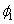and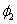the TDSE reads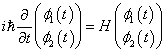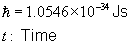where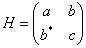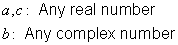is the Hamiltonian describing the system. The solution of this equation gives a complete description of the time evolution of the quantum system. For instance, the probability to find the system in state 1 at time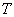is given by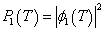The Hamiltonian for a particle in an electromagnetic potential is given by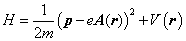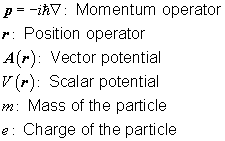The quantum state of the particle is characterized by the amplitude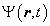for any point in space and time. This amplitude is also called the wave function of the particle. As before, the TDSE governs the time evolution of the wave function. The probability to find the particle at the position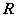at timeis given by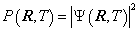The wave function contains all the information about the quantum system. Once it is known for all points in space and time, any physical quantity can be calculated.